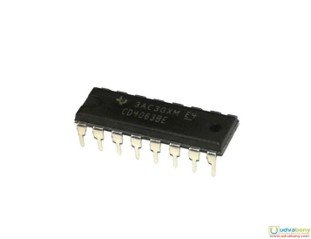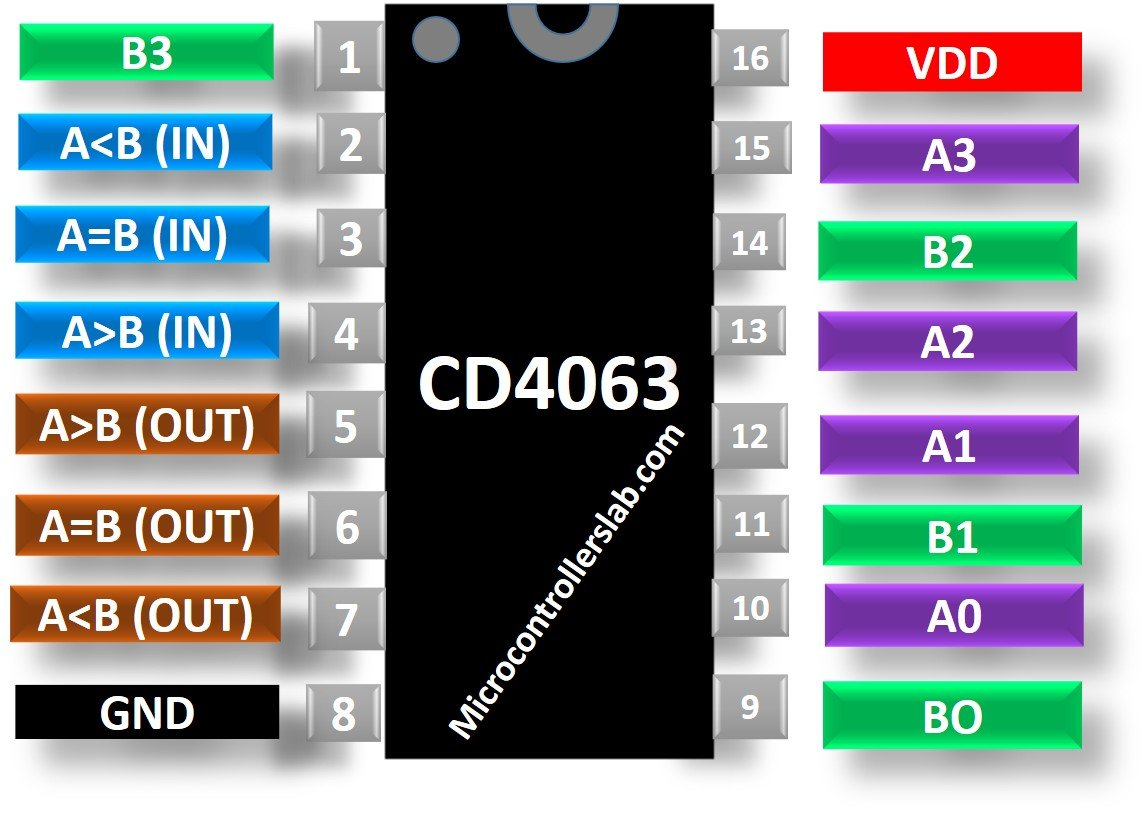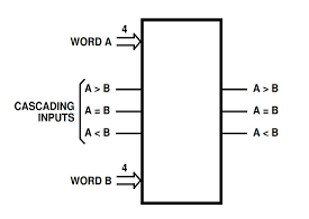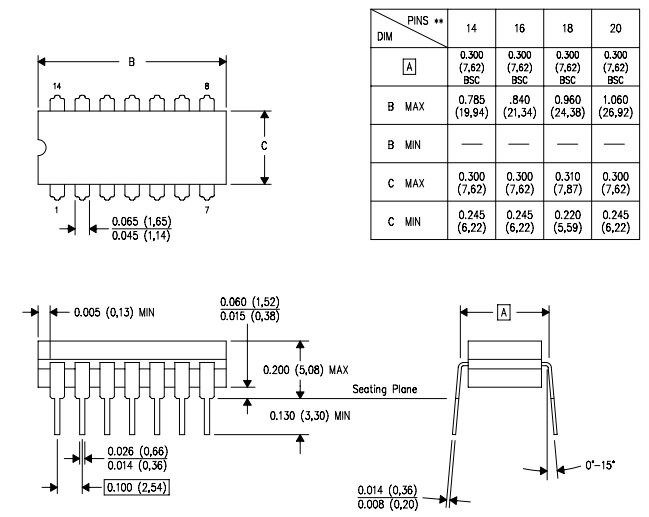# CD4063 4-bit magnitude Comparator IC

CD4063 belongs to a 4000 series of integrated chips. This series (CD4000) is more versatile and consumes lower power as compared to the 4000 series of TTL family. However, their slower speed as compared to TTL limits their applications to the designs which require slow speed.  CD4063 is a 4-bit magnitude comparator which compares two 4 bit numbers and determines whether the first number is greater, less or equal to the second number. For words longer than 4-bits, many CD4063 devices can be cascaded to compare bigger words. It is a digital CMOS comparator used in CPU’s and logic circuits for a wide variety of applications.## Pinout Diagram CD4063

At the sart, this pictures shows the pinout diagram of CD4063 4-bit magnitude Comparator IC and Pin Configuration details.### Pin Configuration Description

Although, CD4063 has 16 pins in total.  But each pin and its function are described below:

• A0, A1, A2 and A3 are the 4 bits of first number.
• B0, B1, B2 and B3 are the 4-bits of second number.
• Pins 5, 6 and 7 are three outputs which tells whether the number A is greater, less or equal to the number B.
• Pins 2, 3 and 4 are inputs for cascading more than one IC. We can use it to compare the numbers that are greater than 4-bits. In other words, we can use these pins to expand the comparaing functionality.
• VDD and VSS is the positive and negative supply voltage.

## CD4063 Comparator Features

• It can compare numbers of 8 bits, 12 bits,16 bits etc by cascading of comparators.
• 4-bit comparator performs its operation at medium speed. In case of 4-bit words, it can take typically 250ns at 10V.
• 100% tested for quiescent current at 20 V.
• Maximum Input Current of:
• 1 µA at full temperature range
• 100 nA at 1 25°C.
• Standardized symmetrical output.
• Parametric ratings are 5V, 10V and 15V.
• For full package temperature range
• Noise margin = 1 V at VDD = 5 V,  for 2 V at VDD = 10 V
• Noise margin = 2.5 V at VDD = 15 V.

CD4585, 74LS85

## How and Where to use it

If you are comparing 4-bit words only, then the cascading inputs of the comparator use in these settings:

1. Pin 2 (A<B) = LOW
2. Pin 3 (A=B) = HIGH
3. Pin 4 (A>B) = LOWIn this way, we will use it as a single IC to compare 4-bit numbers only.

Although, in this modern age, all data processing is atleast in 8-bit or 16-bit. Therefor, If you want to compare large BCD or binary numbers, then this can be achieved by cascading multiple units through these cascading inputs.

Additionaly, Cascading is done by connecting least significant comparator outputs to the corresponding inputs of more significant comparator. The cascading inputs of the least significant comparator are connected in the same way as described for comparison of 4-bit numbers. For example, you want to compare two 8-bit words. The diagram given below shows to connect the two comparators for comparing two 8-bit words.### Comparator Truth Table

This truth table depcits relation of inputs, cascading inputs and output of comparator.### Examples in digital Circuits

Digital Comparators perform a wide variety of arithmetic operations. They can be used for comparing two voltages by comparing an input voltage with a reference voltage. Hence, they can be used in Analogue to Digital convertors. In addition to that, they are also used in control applications to compare numbers which are representing variables like temperature etc. Then the outputs are used to drive the actuators to make the physical variables closest to the reference value. These are used in the decoding circuitry in computers for checking addresses of instructions and microprocessors to select a specific device for the data storage.

## CD4063 Applications

It had a wide variety of applications. Some of them are:

• It is used in controlling servo motors. Servo motors contain a DC motor connected to a potentiometer, gears and a control circuitry. Comparator is used to compare the PWM signal with the reference signal of potentiometer and generates error signal. This error signal causes the rotation of motor.
• CD4063 can be used to compare a reference value with output and thus it is used in process controllers.
• Password verification can be done through this IC by comparing the value of password entered with the password stored in the database.
• One of its major applications is its use in Biometric applications.

### DataSheet